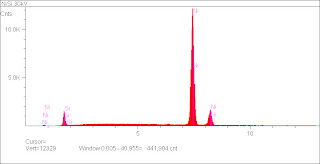## Tuesday, December 6, 2011

### Quantitation with Standards: NiSiEDS quantitative analysis is based on k-ratio's, the ratio of the intensity of a specific element in a mixture to that of a standard of the pure element. In this example of EDS of NiSi at 30kV, there are two k-ratio's, k(Ni Kα) = I(Ni Kα)/I*(Ni Kα) and k(Si Kα) = I(Si Kα)/I*(Si Kα) where the standard data is indicated by a "*". These k-ratio's are at the heart of the ZAF matrix corrections as the matrix corrections are iteratively applied to the k-ratio's until the corrections converge upon a result.

It often thought that the primary source of error in EDS quantitation are the ZAF matrix corrections themselves. While it is true that the ZAF matrix corrections are deficient under certain types of conditions-- a binary compound like NiSi at 30 kV is not one of them.

EDS quantitation can be performed with and without standards. When performed without standards, the standard portion of the k-ratio's is theoretically modeled from X-ray excitation cross-sections. In this example standardless quant yields Si 9.7% and Ni 90.3% (weight%). The expected values are Si 19.3% and Ni 80.7%. The silicon signal is profoundly underestimated.

Why is this so? It's not the ZAF matrix corrections. The theoretically modeled standard data does not account for systematic analytical errors-- in this case the most important being absorption in the detector. Over time the detector window builds up a layer of cryopumped organic material and water from the vacuum chamber. This layer attenuates lower energy X-rays more than higher energy X-rays-- thus tending towards an underestimation of light elements. In the current example Si Kα is at 1.739 keV while Ni Kα is at 7.470 keV.

One possibility is to clean the EDS detector window. While that is possible, it takes some time to totally warm the EDS detector and there is always the possibility of breaking the window.

The other possibility is to run standards. Standards of pure Si and Ni were run at the same beam energy and working distance as the unknown. This data was defined as a standard of known elemental composition and then used to generate calibration data. This calibration data is then used to calculate the k-ratio's using experimental data, in which case the effects of systematic instrumental errors such as window absorption are accounted for.

Repeating the example using standards one gets Si 17.7% and Ni 82.4%-- a drastic improvement. We are still a few weight% from the standard values, but at this point we are close enough to start examining other nuances of the quant process.

The point of the example: standardless quant is, in general, qualitative at best.